# Drawing scatterplots¶

The `incenp.plotting.scatterplot` module provides a `scatterplot` function to facilitate the creation of scatter plots.

Note that what I call a ”scatter plot” here may not be the most common acceptation of the term. I do not mean the 2-dimensional plotting of two variables (one on the x-axis, the other on the y-axis). Rather, I mean the plotting of a single variable on the y-axis, akin to a bar chart, but with all data points depicted as scattered dots.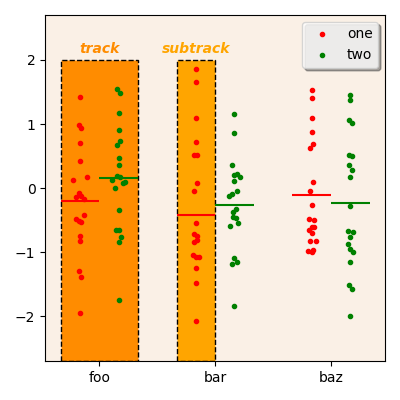A sample scatter plot.

The figure above is a sample “scatter plot”. The orange boxes are not part of the plot, but have been added to illustrate what are tracks and subtracks in the context of the `incenp.plotting.scatterplot` module.

## Sample data¶

The module is intended to work with indexed DataFrame objects (including multi-indexed DataFrame). Let’s create such an object, which we will use throughout this page:

```index = pd.MultiIndex.from_arrays([
['foo'] * 40 + ['bar'] * 40 + ['baz'] * 40 + ['qux'] * 40,
['one', 'two'] * 80
],
names=['first', 'second']
)
df = pd.DataFrame(np.random.randn(160,4), index = index,
columns=['A', 'B', 'C', 'D'])
```

This creates a DataFrame with 4 columns (`A` to `D`) and 160 rows, indexed in two levels (level `first`, with 4 distinct values `foo`, `bar`, `baz`, and `qux`; and level `second`, with 2 distinct values `one` and `two`).

## Quick start¶

As an initial example, here is the call to `scatterplot` to draw the graph above (`ax` is supposed to be a matplotlib.axes.Axes object):

```scatterplot(ax, df, columns='A',
tracks=['foo', 'bar', 'baz'], trackname='first',
subtracks=['one', 'two'], subtrackname='second')
ax.legend(['one', 'two'])
```

The `columns` parameter indicates that the values to be plotted comes from the column named `A`.

The `tracks` parameter gives the index values used to distribute the values of column `A` into three different tracks (one track for rows with index value `foo`, one track for rows with index value `bar`, and so on); the associated `trackname` parameter indicates which index level to use to lookup the values specified in the previous parameter, if `df` is a multi-indexed DataFrame.

The `subtracks` and `subtrackname` parameters are similar to the `tracks` and `trackname` parameter above, but for subtracks instead of tracks. Here, they are used to say that values from rows with index value `one` are to be plotted on one subtrack, while values from rows with index value `two` are to be plotted on another subtrack.

## Playing with tracks, subtracks, columns¶

The following code will plot the same values as above, but will invert the tracks and the subtracks: the second-level index (`second`) will be used to distribute values along tracks while the first-level index (`first`) will be used to distribute values along subtracks:

```scatterplot(ax, df, columns='A',
tracks=['one', 'two'], trackname='second',
subtracks=['foo', 'bar', 'baz'], subtrackname='first')
ax.legend(['foo', 'bar', 'baz'])
```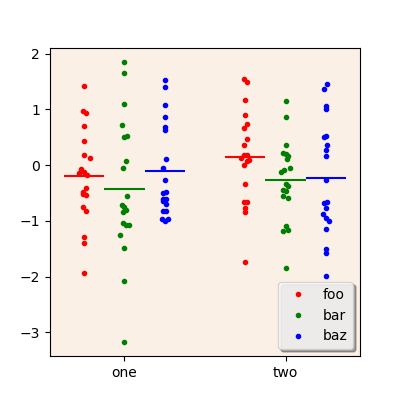A scatterplot with inverted tracks and subtracks.

Values from several columns in the source DataFrame can be plotted at once, by giving a list of column names (instead of a single name) to the `columns` parameter. By default, values from each column are plotted in a different track. In the following examples, values from the columns `A`, `B`, and `C` are plotted; the first-level index is used to distribute values along three different subtracks; the second-level index is used to filter the DataFrame prior to plotting so that only rows with the index value `one` are plotted.

```scatterplot(ax, df.xs('one', level='second'),
columns=['A', 'B', 'C'],
subtracks=['foo', 'baz', 'qux'], subtrackname='first')
ax.legend(['foo', 'baz', 'qux'])
```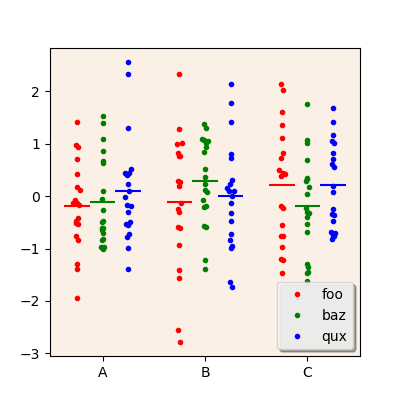A scatterplot with values from several columns of the source DataFrame.

To plot values from several columns as different subtracks rather than different tracks, use the `subtrackcolumns` parameter as in the example below. The `tracks` and `trackname` parameters may then be used to define what goes into the tracks.

```scatterplot(ax, df.xs('one', level='second'),
columns=['A', 'B', 'C'], subtrackcolumns=True,
tracks=['foo', 'baz', 'qux'], trackname='first')
ax.legend(['A', 'B', 'C'])
```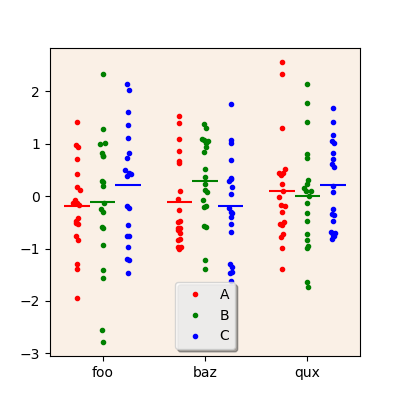A scatterplot with values from several columns of the source DataFrame, plotted as separate subtracks.

## Miscellaneous features¶

When plotting two subtracks, the `testfunc` parameter may be used to have the `scatterplot` function draws the result of a statistical test comparing the values from each subtrack in each track.

The value of the `testfunc` parameter should be a function accepting two DataSeries and returning a P-value, such as a the following wrapper around Scipy’s `mannwhitneyu` function:

```from scipy.stats import mannwhitneyu

def do_mannwhitney(a, b):
result = mannwhitneyu(a, b)
return result.pvalue
```

Below is an example of using such a wrapper, with the resulting plot:

```scatterplot(ax, df, columns='B',
tracks=['foo', 'baz', 'qux'], trackname='first',
subtracks=['one', 'two'], subtrackname='second',
testfunc=do_mannwhitney,
colors='cm')
ax.legend(['one', 'two'])
```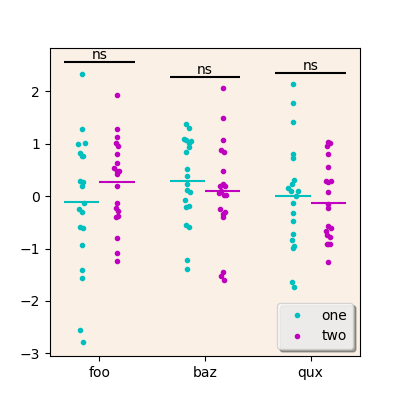A scatterplot with results of statistical tests between subtracks.

The example above also shows the `colors` parameter, used to change the colors for the different subtracks. It can either be a string containing one-letter color codes, or a list of Matplotlib colors. The string or the list must be at least as long as the number of subtracks to plot.